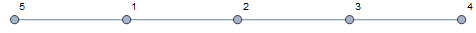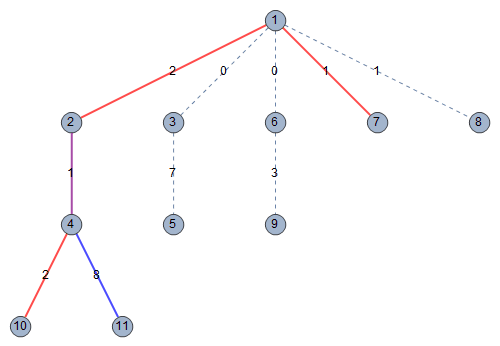#397. 【NOI2018】情报中心

C 国和 D 国近年来战火纷飞。

样例一

input

2
5
1 2 1
2 3 3
3 4 2
1 5 8
2
1 4 5
3 5 8
5
1 2 1
2 3 3
3 4 3
1 5 9
2
1 5 5
2 3 8



output

1
F



explanation• 对于第一组数据，方案一中的节点 $1$ 到节点 $4$ 的最短路径为 $1 \rightarrow 2 \rightarrow 3 \rightarrow 4$，方案二中的节点 $3$ 到节点 $5$ 的最短路径为 $3 \rightarrow 2 \rightarrow 1 \rightarrow 5$。选择这两种方案需要花费 $5 + 8 = 13$ 的代价，并且每一条边的情报都被收集从而得到 $1 + 3 + 2 + 8 = 14$ 的收益，因此总收益减去总代价为 $14 − 13 = 1$。
• 对于第二组数据，方案一中的节点 $1$ 到节点 $5$ 的最短路径为 $1 \rightarrow 5$，方案二中的节点 $2$ 到节点 $3$ 的最短路径为 $2 \rightarrow 3$。这两种方案收集情报的范围没有公共的边，因此非法，所以这组数据不存在合法方案，应输出 F

样例二限制与约定

1$2$$3保证 210$$30$保证
3$200$$300保证 410^3$$2,000$保证$a_i = b_i - 1$
5$10^4$$3 \times 10^4保证a_i = b_i - 1 65 \times 10^4$$10^5$保证$a_i = b_i - 1$
7$10^4$$3 \times 10^4保证c_i=0 85 \times 10^4$$10^5$保证$c_i=0$
9$5 \times 10^4$$10^5保证c_i=0 1010^4$$n$保证$S_1$
11$5 \times 10^4$$n不保证S_1 125 \times 10^4$$n$不保证$S_1$
13$10^4$$3 \times 10^4保证S_2 1410^4$$3 \times 10^4$保证$S_2$
15$5 \times 10^4$$10^5不保证S_2 165 \times 10^4$$10^5$不保证$S_2$
17$10^4$$3 \times 10^4保证 185 \times 10^4$$ 10^5$保证
19$5 \times 10^4$$10^5不保证 205 \times 10^4$$ 10^5$不保证

• 特殊性质 $S_1$：对于任意 $i, j$，保证 $x_i$ 到 $y_i$ 的最短路径所经过的编号最小的节点不同于 $x_j$ 到 $y_j$ 的最短路径所经过的编号最小的节点；
• 特殊性质 $S_2$：对于任意 $i$，保证 $x_i$ 到 $y_i$ 的最短路径所经过的编号最小的节点为节点 $1$。